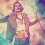## Saturday, October 22, 2011

### Number sequence questions on tests

IQ or aptitude tests, the sort of things you end up writing when you're in school, often feature number sequence questions where you have to fill in the next number in sequence. There's a simple trick that makes an enormous number of them much easier, that I learned when I was quite young and always make a point of teaching to children that I know. It's just applying successive differences. So, for example,
```1  4  9  16 25 ...
3  5  7  9 ```
or
```16  22  34  58 106 ...
6  12   24  48```
Mathematically, for any polynomial taking the differences reduces the degree of the polynomial, which for most of the polynomials you're likely to encounter in test contexts will reduce to a constant in a couple within two or three iterations.

So polynomials can be done mechanically, but the technique works better than that. A fibonacci sequence reduces to itself
```1  1  2  3  5  8 ...
0  1  1  2  3 ```
and so do exponentials, with any constant or polynomial terms reducing as they would have on their own
```1   2   4   8   16   32 ...
1    2   4   8    16```
and in general, sequences that aren't very tricky rapidly become obvious. I think I remember learning about this trick by reading a Mathematical Games column that talked about a computer program designed to take IQ tests that only knew how to do number sequences and shape similarities.

To make sure I haven't been deluding myself and needlessly bothering young relatives I found a set of questions at fibonicci.com and ran through them all with that method. These were the ones ranked as medium difficulty. There were 13 questions, and there were 3 of them that this technique didn't get.

The trickiest one that it did get was
```7  21  14  42  28 ...
14  -7  28  -14 ```
so two alternating sequences, each doubling. Or at least that's the interpretation I took, and it agreed with the test writers. There are an infinite number of patterns that fit any particular sequence, so agreeing with the test writer as to the simplest one is the important criteria. I'm sure there's a point to be made about such tests there.

The sequences it didn't get were
```75  15  25   5  15...
-60  10  -20  10```
which I wasn't sure of the proper answer to, but the internet suggests an alternating series of divide by 5 and add 10.
```1   2   6  24  120...
1   4  18  96
3   14  78```
where each term is the previous term multiplied by n. And
```183  305  527  749  961...
222  222  222  212 ```
and where the trick is not consider the numbers as integers but rather to consider each digit separately, so the next term is 183 again as all the digits wrap back around to their original values.

Obviously there are a lot of more complex sequences that this technique won't help with, but for test questions it's extraordinarily useful.

1.This one bugged me (though I implemented something similar recently in Smalltalk)

111 108 ? 81 72 63

2.Having just completed this test, I thought I'd share my solution for the sequence:

75 15 25 5 15...

I took them as three groups of two digits:

75/5=15, 25/5=5, & 15/5=3

... or have I hideously misunderstood the test? (as an archaeologist with a liberal arts background, this is quite a possibility)!